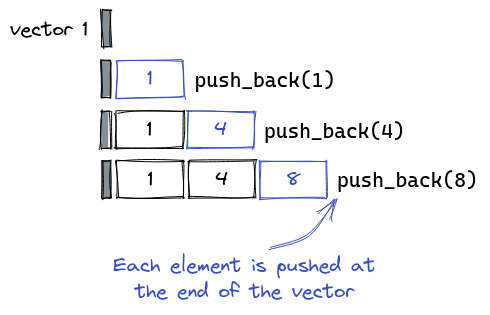# C++ Vector SimulationraihatneloyReplay of Victory Day JU...
Limits 2s, 512 MB

Array is an important data structure in computer science. It is also very important when it comes for problem solving. Very few problems are there all around the online judges in which you can skip using array. But somehow, now-a-days we really can avoid using array because of the great invention in C++: vector.So, why vector has made revolution in problem solving? Because, it can perform dynamic actions. For example, if you have a vector of size 10, but suddenly you need to store one extra element in the vector, you can easily do that by calling a simple function push_back(). Again, you can insert a value at a specific index dynamically in the vector, whereas in array, you have to write your own code using loops and something extra to make this happen.

So, let’s do something with this vector today? We will be given $N$ vectors. Each vector can store one or more integers. Initially the first vector will only contain integer 1, 2nd vector will only contain integer 2, 3rd vector will only contain integer 3, …, $N$-th vector will only contain integer $N$.

We will have to perform following two tasks:

1. Update operation: You will be provided two integer numbers $X$ and $Y$ ($X \ne Y$). Let $A$-th vector contain the number $X$ and $B$-th vector contain the number $Y$. You have to merge these two vectors into a single vector. As a result $A$-th and $B$-th vector will no longer exist and a new vector will be produced containing all the element from both $A$-th vector and $B$-th vector. If $X$ and $Y$ are already in the same vector, there will be no effect.
2. Query operation: You will be provided a single integer $X$. Let $A$-th vector contain the number $X$. This $A$-th vector can contain a lot of other numbers too. You will have to find the median of those numbers which are contained by the $A$-th vector.

I already tried to code this thing. But alas! I failed ☹️. Don’t know how to solve it. Can you please help me out?

## Input

Each file of input will start with a single integer $T$ ($1 \le T \le 5$), the number of test cases. Each test case starts with a line having two integers, $N$, $M$ ($1 \le N, M \le 100000$). $N$ represents the number of initial vectors. $M$ represents the number of tasks we have to complete. Each of the next $M$ lines will contain any of the following two types of task:

• 1 X Y - This type of input means to perform task of 1st type. ($1 \le X,Y \le N, X ≠ Y$)

• 2 X - This type of input means to perform task of 2nd type.

## Output

For each test case you will have to print a line with the case number.

After that, for every 2nd type of task, you will just need to print the median of the selected vector. For avoiding any precision error, you will have to print the answer multiplied by 2.

## Sample

InputOutput
1
5 5
1 2 3
2 2
1 3 5
1 1 5
2 2

Case 1:
5
5


Initially there are 5 vectors: $$, $$, $$, $$, $$.

After 1st task: $$, $[2, 3]$, $$, $$

Output of 2nd task: 5 (As the vector contains $[2, 3]$)

After 3rd task: $$, $[2, 3, 5]$, $$

After 4th task: $[1, 2, 3, 5]$, $$

Median is the middle of a sorted list of numbers. Median of $[1,2,3]$ is 2 and median of $[1,2,3,4]$ is 2.5.uDebug

### Submit